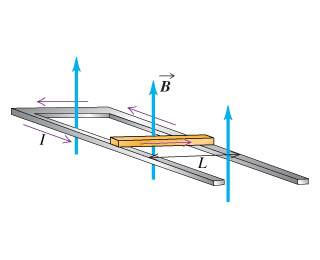# Rail Guns and acceleration

## Homework Statement

A conducting bar with mass=25.0 kg and length L=51.0 cm slides over horizontal rails that are connected to a voltage source. The voltage source maintains a constant current I= 2400A in the rails and bar, and a constant, uniform, vertical magnetic field B = 0.480 T fills the region between the rails.
See figure:It has been suggested that rail guns based on this principle could accelerate payloads into earth orbit or beyond. Find the distance the bar must travel along the rails if it is to reach the escape speed for the earth (11.2 km/s).
For simplicity, assume the net force on the object is equal to the magnetic force, as in parts A and B, even though gravity plays an important role in an actual launch into space.

## Homework Equations

I know the magnetic force is 588N.
F=ma
V = at
X = at^2

## The Attempt at a Solution

588/25 = 23.52 m/s^2
11200 m/s = 23.52 m/s^2 * t
t = 476.190

x = 23.52 m/s^2 * 476.19^2
5333322.666672 m

Am I missing something, it says that is incorrect....